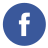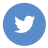### java BigDecimal

java

In Java, the `BigDecimal` class is a high-precision decimal data type that is used to represent numbers with a large number of digits or with a high degree of precision. It is part of the `java.math` package and can be used to perform arithmetic operations and compare numbers with a high degree of accuracy.

Here is an example of how to use the `BigDecimal` class in Java:

``````import java.math.BigDecimal;

public class Main {
public static void main(String[] args) {
// Create a BigDecimal from a string
BigDecimal x = new BigDecimal("0.1");
BigDecimal y = new BigDecimal("0.2");

// Perform arithmetic operations
BigDecimal difference = x.subtract(y);
BigDecimal product = x.multiply(y);
BigDecimal quotient = x.divide(y);

// Print the results
System.out.println(sum); // prints 0.3
System.out.println(difference); // prints -0.1
System.out.println(product); // prints 0.02
System.out.println(quotient); // prints 0.5
}
}``````

This code creates two `BigDecimal` objects and performs arithmetic operations on them using the `add()`, `subtract()`, `multiply()`, and `divide()` methods. It then prints the results to the console.

For more information on the `BigDecimal` class and how to use it in Java, you can refer to the Java documentation: